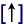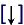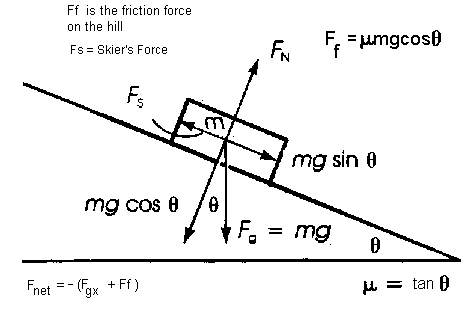### Free Body Diagrams (F.B.D.) on Inclines

A free body diagram is a simple sketch showing all the forces acting on an object when it is on its own (i.e. removed from its surrounding).

Here are some guidelines for drawing Free Body Diagrams.

• Forces are represented by vectors
• A relative scale should be used to show the relative size of the forces whenever possible.
• Only the forces acting on the object should be included not the forces that the object exerts on other objects.
• All forces (vectors) should be drawn with respect to the center of gravity (or center of mass of the object).
• This point is usually located at the geometric center of the object

#### A. FBD for an object resting on a flat (horizontal) surfaceWhere:
Fis the elastic force pointing up
Fis the force of gravity; pointing down

• Note the symbols used to represent the forces Fe is the elastic Force, Fg is the force of gravity.
• Note also the arrow head on top of the symbol indicating that the Force is a vector.
• Note the direction of the forces; Fe is upwhile Fg is down.• We say that these forces acting collinearly (in the same line of action)  are equal in magnitude and opposite in direction are in static equilibrium.
• When this happens the forces on an object are said to be balanced.

#### B. FBD for an object resting on an inclined plane

In the diaram below, an object with mass m rests on an incline.
Here the Normal Force Fstill points as predicted but notice that it is alwyas perpendicular to the plane where the object rests.
The force of gravity F  points straight down ytowards the centre of the Earth, as redicted.
However, it is  the y component of the force of gravity that counterbalances the normal force collinearly.
The magnitude of the components of the force of gravity component can be calculated by trigonometric rules.